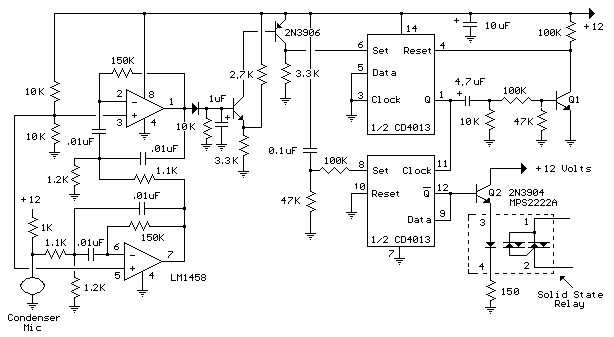# Whistle On - Whistle Off Electronic Schematics > Power > Whistle On - Whistle Off

This is an extension of the CMOS toggle flip flop circuit shown in the "Circuits controlling relays" section with the addition of two bandpass filters and condenser microphone so the relay can be toggled by whistling at it. The condender mic used is a PC board mount Radio Shack #270-090C. The filters are tuned to about 1700 Hz, or the third Ab above middle C on a piano keyboard which is a fairly easy note for me to whistle. Resistor values for the filter can be computed using the three formulas below but we need to assume a gain and Q factor for the filter and the Q of the circuit must be greater than the square root of (Gain/2). The microphone produces only a couple millivolts so the overall gain needs to be around 4000 or around 65 for each filter. The Q or quality factor is the ratio of the center frequency to the bandwidth (-3dB points) and was chosen to be 8 which is greater than 5.7 which is the minimum value for a gain of 65. Both capacitor values need to be the same for easy computation of the resistor values and were chosen to be 0.01uF which is a common value and usable at audio frequencies. From those assumptions, the resistor values can be worked out from the following formulas.

R1 = Q/(G*C*2*Pi*F) = 8/(65*.01^-6*6.28*1700) = 1152 or 1.1K
R2 = Q / ((2*Q^2)-G)*C*2*Pi*F) = 8/((128-65)*.01^-6*6.28*1700)= 1189 or 1.2K
R3 = (2*Q)/(C*2*Pi*F) = 16/(.01^6*6.28*1700) = 150K

The op-amps are biased using a voltage divider of two 10K resistors so the output will be centered around half the supply voltage or 6 volts. The output of the second filter charges a 1uF cap at the base of a NPN transistor (2N3904 or similar). The emitter voltage is biased at 6.6 volts using the 3.3K and 2.7K resistors so that the transistor will conduct and trigger the flip flop when the peak signal from the filter reaches 8 volts. The 8 volt figure is the emitter voltage (6.6) plus the emitter base voltage drop (0.7) plus the diode drop (0.7). The sensitivity can be adjusted by changing the value of either the 2.7K or 3.3K resistors so that more or less signal amplitude is needed to trigger the flop flop.Whistle On - Whistle Off circuit

Title: Whistle On - Whistle Off
electronic circuit
Source: unknown
Published on: 2007-07-21
Print version: